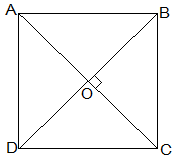# ABCD is a rhombus. Show that diagonal AC bisects ∠A as well as ∠C and diagonal BD bisects ∠B as well as ∠D.

Given that,

ABCD is a rhombus.

AC and BD are its diagonals.

To Prove

AC bisects ∠A as well as ∠C and diagonal BD bisects ∠B as well as ∠D.

Solution:As, ABCD is a rhombus, so

AB = BC = CD = DA

∠ADB= ∠ABD……………………….(1) [Angles opposite to equal sides of a triangle are equal]

∠ADB = ∠CBD …………………..(2) [ ∵ Alternate interior angles are equal] (Rhombus is a parallelogram)

From (1) and (2), we have

∠CBD = ∠ABD …………………..(3)

∠ABD= ∠CDB ……………………..(4) [ ∵ Alternate interior angles are equal]

From (1) and (4),

Hence, BD bisects ∠B as well as ∠D.

Similarly, we can prove that AC bisects ∠C as well as ∠A.

Hence Proved(18)(10)